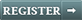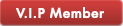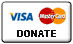## Using v1040 maps without metas available!function(d,s,id){var js,fjs=d.getElementsByTagName(s);if(!d.getElementById(id)){js=d.createElement(s);js.id=id;js.src="//platform.twitter.com/widgets.js";fjs.parentNode.insertBefore(js,fjs);}}(document,"script","twitter-wjs"); //<![CDATA[ document.write('<fb:like href="" send="false" layout="button_count" style="position:relative;bottom:6px;" show_faces="false" action="like"></:like>'); //]]>

### Using v1040 maps without metas available

Special v1040 maps

Activation of maps with equivalent metas. If you have a map that has no meta, you may be able to activate it if there is an equivalent meta.

So, in simple terms (Iberia_1040.9849 example):

Download/decompress/copy the map that you want to activate (but for which you don't have the meta).
Delete the relavent .meta file
Copy into the map folder the .meta file from the other map (same country - compatible as number) for the same country
Activate the map with the usual system.

For example:

Copy map Iberia_1040.9849
Delete map Iberia_1040.9849 meta file (Iberia-102191.meta)
Copy the meta of Iberia_1040.9805 (Iberia-102147.meta) that has meta code into map Iberia_1040.9849 folder
Unlock the map.

The equivalent numbers are:

• 1040_9799 = 1040_9800 = 1040_9802 = 1040_9852 = 1040_9867 = 1040_9926 = 1041_Truck
• 1040_9804 = 1040_9805 = 1040_9807 = 1040_9849 = 1040_9850 = 1040_9868 = 1040_9923 = 1040_9925
• 1040_9809 = 1040_9810 = 1040_9812 = 1040_9854 = 1040_9856 = 1040_9857 = 1040_9858 = 1040_9861 = 1040_9869 = 1040_9910 = 1040_9911 = 1040_9914

Very important: only when the size of the meta files that we are going to change are the same
Note: To the man who copies the data, I hope this time he mentions the forum of origin and the author.
Orni offlineJoined: Tue May 03, 2011 11:35 am
Posts: 39
Has thanked: 15 times
Been thanked: 188 times